# designFracDelayFIR

Design band-limited fractional delay FIR filter

## Syntax

``h = designFracDelayFIR(fd)``
``h = designFracDelayFIR(fd,N)``
``h = designFracDelayFIR(fd,TBW)``
``[h,i0] = designFracDelayFIR(___)``
``[h,i0,MBW] = designFracDelayFIR(___)``

## Description

````h = designFracDelayFIR(fd)` designs a fractional delay FIR filter of delay `fd`, default length of `50`, approximate bandwidth of 0.9.```

example

````h = designFracDelayFIR(fd,N)` designs a fractional delay FIR filter of delay `fd` and length `N` > 1. The function designs the filter to achieve the specified FIR length.```

example

````h = designFracDelayFIR(fd,TBW)` designs a fractional delay FIR filter of delay `fd` and a combined bandwidth of at least `TBW`, where `TBW` < `0.999` and is specified in normalized frequency units. The function automatically determines the appropriate FIR length N for which the combined bandwidth is at least `TBW`.```
````[h,i0] = designFracDelayFIR(___)` returns the integer latency of the FIR filter. This value is approximately `N`/2. The nominal group delay of the filter is `i0`+`fd`.```

example

````[h,i0,MBW] = designFracDelayFIR(___)` returns the measured combined bandwidth of the filter `h`.```

## Examples

collapse all

Design a fractional delay FIR filter using the `designFracDelayFIR `function. Pass the delay and the filter length as the input arguments to the function. Vary the filter length and observe the effect on the measured combined bandwidth and the nominal group delay.

Vary Filter Length

Filter Length Set to 8

Specify the delay to be 0.25 and the filter length to be 8 and design the fractional delay FIR filter.

```fd = 0.25; len = [8 32 64]; [h1,i10,bw1] = designFracDelayFIR(fd,len(1))```
```h1 = 1×8 -0.0086 0.0417 -0.1355 0.8793 0.2931 -0.0968 0.0341 -0.0074 ```
```i10 = 3 ```
```bw1 = 0.5810 ```

The nominal group delay of the filter `i10+fd` equals 3.25 samples. The measured combined bandwidth of the filter is 0.5810 in normalized frequency units.

Filter Length Set to 32

Repeat the process with a filter length of 32 taps.

`[h2,i20,bw2] = designFracDelayFIR(fd,len(2))`
```h2 = 1×32 -0.0001 0.0004 -0.0009 0.0017 -0.0029 0.0046 -0.0071 0.0104 -0.0148 0.0208 -0.0291 0.0410 -0.0594 0.0926 -0.1752 0.8983 0.2994 -0.1252 0.0758 -0.0515 0.0367 -0.0266 0.0193 -0.0139 0.0098 -0.0067 0.0044 -0.0028 0.0016 -0.0009 0.0004 -0.0001 ```
```i20 = 15 ```
```bw2 = 0.8571 ```

The nominal group delay of the filter now equals 15.25 samples. By increasing the filter length, the integer latency `i0` also increases, resulting in an increase in the nominal group delay. The combined bandwidth of the filter has increased to 0.8571 in normalized frequency units.

Filter Length Set to 64

Increase the filter length to 64 taps. The group delay increases to 31.25 samples, and the integer latency is 31 samples. The measured combined bandwidth of the filter further increases to 0.9219. That is a bandwidth coverage of 92.19% of the overall bandwidth. As the filter length continues to increase, the combined bandwidth tends closer towards 1.

`[h3,i30,bw3] = designFracDelayFIR(fd,len(3))`
```h3 = 1×64 -0.0000 0.0001 -0.0001 0.0002 -0.0003 0.0004 -0.0006 0.0008 -0.0010 0.0013 -0.0017 0.0022 -0.0027 0.0034 -0.0042 0.0051 -0.0061 0.0074 -0.0088 0.0105 -0.0125 0.0149 -0.0177 0.0212 -0.0255 0.0311 -0.0386 0.0494 -0.0664 0.0979 -0.1787 0.8997 0.2999 -0.1277 0.0801 -0.0575 0.0442 -0.0352 0.0288 -0.0239 0.0200 -0.0168 0.0142 -0.0120 0.0101 -0.0085 0.0071 -0.0059 0.0049 -0.0040 ```
```i30 = 31 ```
```bw3 = 0.9219 ```

Plot Magnitude Response

Plot the resulting magnitude response of the three filters using `fvtool`. Mark the measured combined bandwidth of the three filters. By increasing the filter length, you can see that the measured combined bandwidth increases.

```hfv = fvtool(h1,1,h2,1,h3); ax = findall(hfv,"type","axes"); hline = findobj(ax(2),'Type','line'); xline(bw1, "LineStyle",'--', "LineWidth",2,'Color',hline(3).Color) xline(bw2, "LineStyle",'--', "LineWidth",2,'Color',hline(2).Color) xline(bw3, "LineStyle",'--', "LineWidth",2,'Color',hline(1).Color) title('Magnitude Responses in dB','FontSize',12) legend('Gain Response (N = 8)','Gain Response (N = 32)','Gain Response (N = 64)',... 'Measured Combined Bandwidth (N = 8)',... 'Measured Combined Bandwidth (N = 32)',... 'Measured Combined Bandwidth (N = 64)','Location','Southwest','FontSize',12);```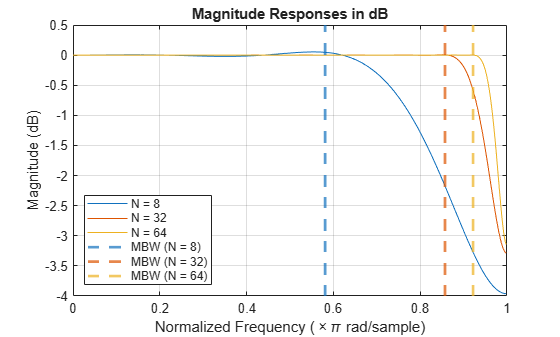Plot Group Delay Response

Plot the group delay response of the three filters using `fvtool`. Mark the nominal group delay i0 + fd of the three filters. By increasing the filter length, you can see that the nominal group delay increases.

```hfv = fvtool(h1,1,h2,1,h3,1,"Analysis","grpdelay"); ax = findall(hfv,"type","axes"); hline = findobj(ax(2),'Type','line'); yline(i10+fd, "LineStyle",'--', "LineWidth",2,'Color',hline(3).Color) yline(i20+fd, "LineStyle",'--', "LineWidth",2,'Color',hline(2).Color) yline(i30+fd, "LineStyle",'--', "LineWidth",2,'Color',hline(1).Color) title('Group Delay Responses','FontSize',12) legend('Group Delay Response (N = 8)','Group Delay Response (N = 32)','Group Delay Response (N = 64)',... 'Nominal Group Delay (N = 8)',... 'Nominal Group Delay (N = 32)',... 'Nominal Group Delay (N = 64)','Location','west','FontSize',12); ax(1).YLim = [-10,40];```Design a fractional delay FIR filter using the `designFracDelayFIR `function. Pass the delay and the combined bandwidth as input arguments to the function.

Specify the delay to be 0.786 and the target combined bandwidth to be 0.8. The function designs a filter that has a length of 22 taps, an integer latency i0 of 10 samples, and a combined bandwidth `m`bw of 0.8044 in normalized frequency units. This mbw value makes the combined bandwidth coverage to be 80.44% of the frequency domain and exceeds the specified target combined bandwidth. The nominal group delay of the filter `i0+fd` equals 10.786.

```fd = 0.786; tbw = 0.8; [h,i0,mbw] = designFracDelayFIR(fd, tbw)```
```h = 1×22 0.0003 -0.0011 0.0026 -0.0052 0.0094 -0.0156 0.0248 -0.0386 0.0611 -0.1052 0.2512 0.9225 -0.1548 0.0769 -0.0455 0.0281 -0.0173 0.0102 -0.0057 0.0028 -0.0012 0.0003 ```
```i0 = 10 ```
```mbw = 0.8044 ```

Plot the impulse response of the FIR.

```stem((0:length(h)-1),h); xlabel('h'); ylabel('h[n]'); title('Impulse Response of the Fractional Delay FIR')```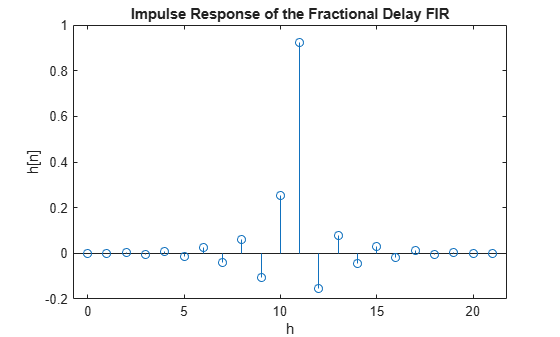Plot the resulting magnitude response and the group delay response using `fvtool`. Mark the nominal group delay and the combined bandwidth of the filter.

```hfv = fvtool(h,'Analysis','magnitude','OverlayedAnalysis','grpdelay'); ax = findall(hfv,'type','axes'); xline(mbw, 'LineStyle',':', 'Color','b', 'LineWidth',2) xline(tbw, 'LineStyle','--', 'Color','m', 'LineWidth',2) yline(ax(1),i0+fd, 'LineStyle',':','Color','r','LineWidth',1) yticks(ax(1),[i0, i0+fd,i0+1:i0+9]); yticklabels(ax(1),'auto') legend('Gain Response','Measured Combined Bandwidth',... 'Target Combined Bandwidth','Group Delay Response','Nominal Group Delay', ... 'Location','west','FontSize',12) grid off```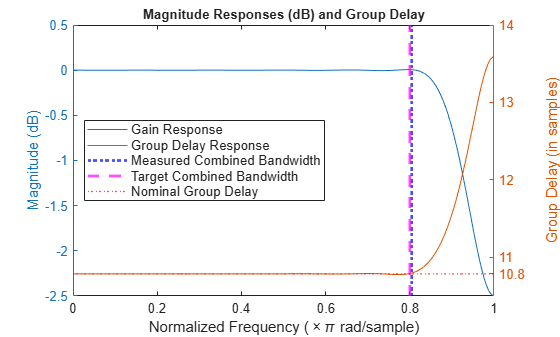Design a fractional delay FIR filter using the `designFracDelayFIR` function. Determine the group delay of the designed filter. Create a `dsp.FIRFilter` object that uses these designed coefficients and hence has the same group delay. Alternately, create a sampled sequence of a known function. Pass the sampled sequence to the FIR filter. Compare the output of the FIR filter to the shifted samples of the known function. Specify this shift to be equal to the group delay of the FIR filter. Verify that the two sequences match.

Specify the delay of the fractional delay FIR filter to be 1/3 and the length to be 6 taps.

```fd = 1/3; len = 6;```

Design the filter using the `designFracDelayFIR` function and determine the center index i0 and the combined bandwidth `bw` of the filter. The group delay of the filter you have designed is `i0` + `fd` or approximately 2.33 for the bandwidth of `bw`.

`[h,i0,bw] = designFracDelayFIR(fd,len)`
```h = 1×6 0.0293 -0.1360 0.7932 0.3966 -0.1088 0.0257 ```
```i0 = 2 ```
```bw = 0.5158 ```

Create a `dsp.FIRFilter` object and set its numerator to the filter coefficients h. This filter is now effectively a fractional delay FIR filter. Verify that the group delay response of this filter is approximately 2.33 for the duration of the bandwidth bw.

```fdf = dsp.FIRFilter(h); grpdelay(fdf)```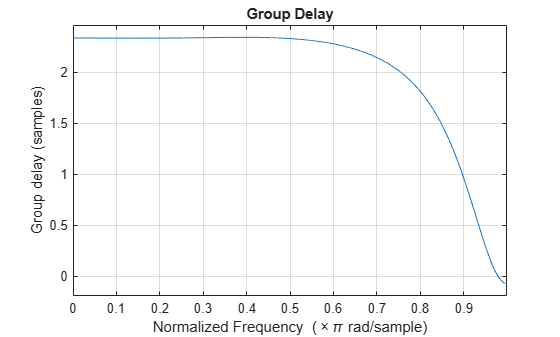Compare with Shifted Function

Define a sequence x as samples of a known function.

```f = @(t) (0.1*t.^2+cos(0.9*t)).*exp(-0.1*(t-5).^2); n = (0:19)'; t = linspace(0,19,512); x = f(n); % Samples```

Plot the sampled values x against the original known function f(t).

```scatter(n,x,20,'k','filled'); hold on; plot(t,f(t),'color',[0.5 0.5 0.5],'LineWidth',0.5) hold off; xlabel('Time') legend(["Input Samples","f(t)"]) title('Input Sequence with Known Underlying Analog Signal') ax = gca; ax.XGrid='on';```Pass the sampled sequence x through the FIR filter. Plot the input sequence and output sequence.

```y = fdf(x); subplot(2,1,1); stem(x); title('Input Sequence'); xlabel('n') subplot(2,1,2) stem(y); title('FIR Output Sequence'); xlabel('n')```Shift the input sequnce horizontally by `i0` + `fd`, which is equal to the group delay of the FIR filter. Plot the function f(t-i0-FD). Verify that the input and output sequences fall roughly on the shifted function.

```figure scatter(n,y,20,'red','filled') hold on; scatter(n+i0+fd,x,20,'black','filled') plot(t,f(t-i0-fd),'Color',[1,0.5,0.5],'LineWidth',0.1) xlabel('Time') legend(["Filter output","Shifted Input Samples","Shifted f(t-i0-fd)"]) hold off grid on title('Input and Output Sequences Aligned and Overlaid')```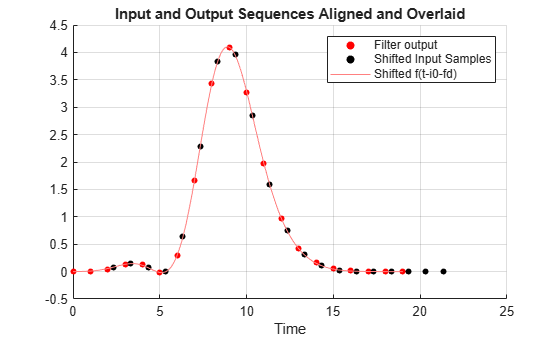## Input Arguments

collapse all

Fractional delay of the filter, specified as a positive scalar in the range [0,1]. The fractional delay value that you specify determines the measured combined bandwidth `MBW` of the filter. When `fd` is set to `0` or `1`, the designed filter has a full bandwidth.

Data Types: `single` | `double`

Length of the fractional delay FIR filter, specified as an integer greater than 1. In order to specify the length of the filter, you must specify the second input argument of the function to be an integer that is greater than 1. The function treats this value as the desired filter length and returns a filter that is N taps long.

Data Types: `single` | `double`

Target combined bandwidth, specified as a positive scalar less than 0.999. This is the value of the combined bandwidth that the function must satisfy. Combined bandwidth is defined as the minimum of the gain bandwidth and the group delay bandwidth.

In order to specify the target combined bandwidth, you must specify the second input argument of the function to be a real scalar that is less than `0.999`. The function then treats this value as the target combined bandwidth, determines the corresponding filter length, and designs the filter accordingly.

Specify a higher target combined bandwidth for a longer filter. For example `TBW` set to 0.9 yields a filter of length of 52. For `TBW` that is set to 0.99, the length is 724. This value is more than 10 times longer. As `TBW` tends towards 1, the filter length theoretically tends towards infinity.

Data Types: `single` | `double`

## Output Arguments

collapse all

Coefficients of the fractional delay FIR filter, returned as a real-valued `N`-length row vector. When fractional delay is the only input to the function, the function designs a filter of length 50. The bandwidth for this default filter is approximately 0.9. When the second input argument is specified, the function designs the filter according to the following:

• When the second input argument to the function is specified to be a value greater than 1, the function treats this value as the desired filter length and returns a filter that is `N` taps long.

• When the second input argument to the function is specified to be a value less than `0.999`, the function treats this value as the desired combined bandwidth `TBW`, determines the corresponding filter length, and designs the filter accordingly.

Data Types: `single` | `double`

Integer latency of the designed FIR filter, returned as an integer value. Integer latency is the smallest integer shift required to make the symmetric Kaiser window causal. This value is approximately equal to half the filter length, N/2. For more details, see Integer latency, i0.

The nominal group delay of the filter is given by `i0`+`fd`.

Data Types: `single` | `double`

Measured combined bandwidth, returned as a real positive scalar that is less than `0.999`. This is the value of the combined bandwidth of the designed filter. Combined bandwidth is defined as the minimum of the gain bandwidth and the group delay bandwidth.

When you specify the second input argument and the value is less than `0.999`, the function treats this value as the target combined bandwidth `TBW`. The function designs the filter such that the measured combined bandwidth `MBW` meets or exceeds the target combined bandwidth `TBW`. The filter length is determined such that this bandwidth constraint is met.

When you specify the second input argument and the value is an integer greater than 1, the function treats this value as the desired length `N` of the filter. The measured combined bandwidth in this case varies with the length you specify. Larger the value of `N`, higher is the measured combined bandwidth `MBW`. See the plot below that shows this variation. As the filter length grows, the combined bandwidth of the filter moves closer towards 1. The red dashed vertical line marks the combined bandwidth for each length. The fractional delay value for each of these filters is set to 0.3Data Types: `single` | `double`

collapse all

### Fractional Delay FIR Filter

The fractional delay FIR filter is an FIR approximation of an ideal sinc shift filter with a specified fractional (non-integer) delay value `fd` within [0,1].

The ideal shift filter models a band-limited D/A interpolator followed by shifted A/D uniform sampling. Assuming uniform sampling rate and shift invariant interpolation, the resulting overall system can be expressed as a convolution filter, approximated by an FIR filter. In other words, $y\left[n\right]={h}_{fd}\left[n\right]\ast x\left[n\right]$, which encapsulates the D/A interpolation, shift, and A/D sampling chain as depicted in the figure.where,

`$\begin{array}{l}{h}_{fd}\left[n\right]=\mathrm{sinc}\left(n-fd\right),\\ \stackrel{^}{x}\left(t\right)=\sum _{k}x\left[k\right]\mathrm{sinc}\left(t-k\right)\\ ⇒\stackrel{^}{x}\left(t+fd\right)=\sum _{k}x\left[k\right]\mathrm{sinc}\left(t+fd-k\right)\end{array}$`

The frequency response of the ideal shift filter is given by the following equation:

`${H}_{fd}\left(\omega \right)={e}^{-j\omega fd}$`

The ideal shift filter has a flat unity gain response, and a constant group delay of `fd`, where `fd` is the fractional delay value you specify.

The FIR approximation is obtained by truncating the ideal filter and weighting the truncated filter by a Kaiser window.

`$\stackrel{^}{x}\left(n+fd\right)\approx y\left[n\right]=\left(h\ast x\right)\left[n\right],\text{ }where\text{ }h\left[m\right]=\mathrm{sinc}\left(m+fd\right)·{K}_{N,\beta }\left[m\right]$`

where, ${K}_{N,\beta }\left[m\right]$ is a Kaiser window of length `N` and has a shape parameter β. The Kaiser window is designed to optimize the FIR frequency response, maximizing the combined bandwidths of both gain response and group delay response.

To make the FIR approximation causal, an additional shift of `i0` is introduced, making the nominal group delay of the filter equal to `i0`+`fd`. The frequency response of the truncated filter is given by, $H\left(\omega \right)={e}^{-j\omega \left(fd+{i}_{0}\right)}$.

For more details, see Integer latency, i0.

### Integer latency, `i0`

Integer latency, `i0`, is the smallest integer shift that is required to make the symmetric Kaiser window causal.

The ideal `sinc` shift filter is an allpass filter, which has an infinite and non-causal impulse response. To approximate this filter, the function uses a finite index Kaiser window of length `N` that is symmetric around the origin and captures the main lobe of the `sinc` function.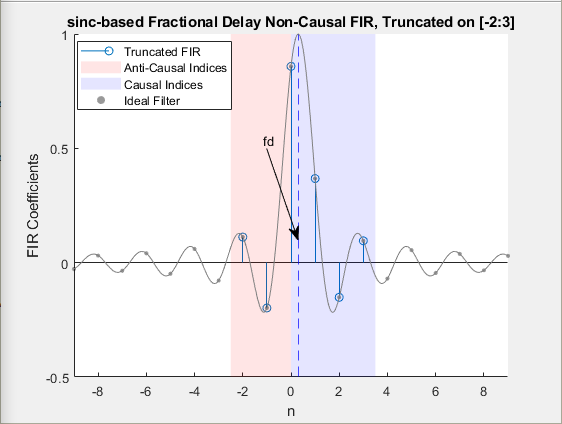Due to the symmetric nature of the window, half of the window (approximately equal to N/2) is on the negative side of the origin making the truncated filter anti-causal. To make the truncated filter causal, shift the anti-causal (negative indices) part of the FIR window by an integer latency, `i0`, that is approximately equal to N/2.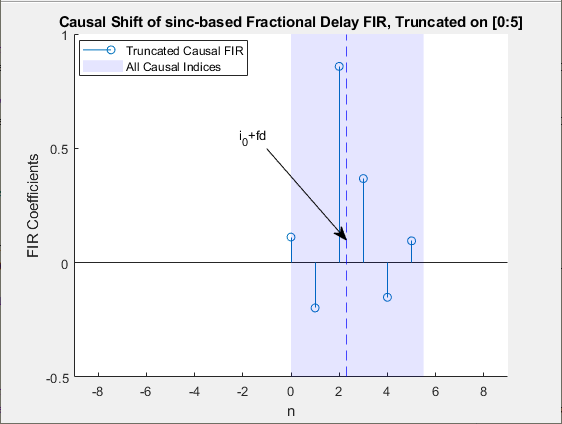The overall delay of the causal FIR filter is `i0`+`fd`, where `fd` is the fractional delay value you specify.

For more details on FIR approximation, see the Causal FIR Approximations of an Ideal sinc Shift Filter section in Design Of Fractional Delay FIR Filters.

### Gain Bandwidth

Given an FIR frequency response H(ω), the gain bandwidth is the largest interval [0 Ba] over which the gain response |H(ω)| is close to 1 up to a given tolerance value, tol.

`${B}_{a}=\underset{\omega }{\mathrm{max}}\left\{||H\left(\nu \right)|-1|`

### Group Delay Bandwidth

Given a tolerance tol and a group delay response G, the group delay bandwidth is the largest interval [0 Bg] such that the group delay is close to the nominal value, `fd`.

`${B}_{g}=\underset{\omega }{\mathrm{max}}\left\{|G\left(\nu \right)-fd-{i}_{0}|`

### Combined Bandwidth

Combined bandwidth is defined as the minimum between the gain bandwidth and the group delay bandwidth.

`${B}_{c}=\mathrm{min}\left({B}_{a},{B}_{g}\right)$`

Combined bandwidth depends on the fractional delay `fd` and the length of the FIR filter `N`.

## Extended Capabilities

### Topics

Introduced in R2021a

## SupportGet trial now